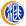### 面值50000元的人民币你见过吗

}500)this.width=500 align=center hspace=10 vspace=10 rel=nofollow/>

500)this.width=500 align=center hspace=10 vspace=10 rel=nofollow/500)this.width=500 align=center hspace=10 vspace=10 rel=nofollow/>

500)this.width=500 align=center hspace=10 vspace=10 rel=nofollow/